# SAT Math Multiple Choice Question 867: Answer and Explanation

### Test Information

Question: 867

12. The average gas mileage for n vehicles on one car lot is 21 miles per gallon. The average gas mileage for p vehicles on another car lot is 25 miles per gallon. When the vehicles on both lotsare combined, the average gas mileage is 22.5 miles per gallon. What is the valueof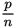?

• A.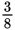• B.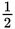• C.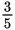• D. 1

Explanation:

C

Difficulty: Hard

Category: Problem Solving and Data Analysis / Statistics and Probability

Strategic Advice: In some questions, you can't find the value of individual variables, but you can find a comparison (or ratio) of the variables. In questions such as these, start with definitions and formulas that you know, and work toward the requested ratio.

Getting to the Answer: The average of a set of values is the sum of the values divided by the number of values. Use the information provided in the question to fill in as much information as you can about the two car lots. You might want to give the lots names, such as Lot A and Lot B:

Lot A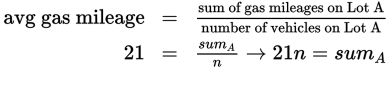Lot B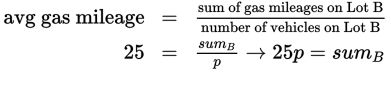Now, put the averages together:

Lot A + Lot B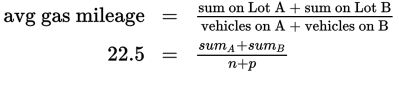It may not seem like you're making progress, but you're actually very close. You're looking for the ratio of p over n, so substitute 21n for sumA (found earlier) and 25p for sumB and simplify the equation by cross-multiplying and combining like terms: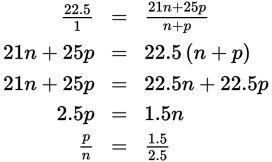To remove the decimals from the fraction, multiply the numerator and dominator by 2 to get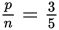, which matches (C).